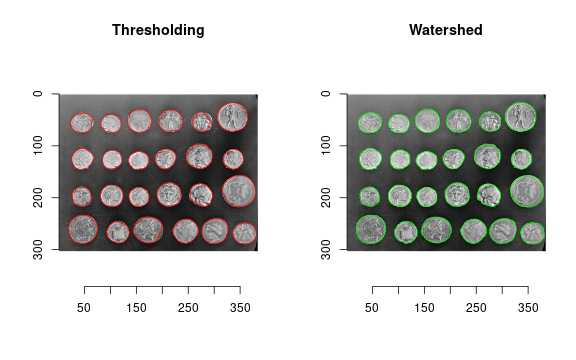If you've already gone through the “Getting started” vignette, you know about image objects (“cimg” class). This vignette introduces pixsets, which is the other important kind of objects. Pixsets represent sets of pixels, or equivalently binary images, AKA “masks”.

``````library(imager)
``````

# From images to pixsets and back

A pixset is what you get if you run a test on an image:

``````im <- load.example('parrots') %>% grayscale
px <- im > .6 #Select pixels with high luminance
px
``````
``````Pixel set of size 68993. Width: 768 pix Height: 512 pix Depth: 1 Colour channels: 1
``````
``````plot(px)
``````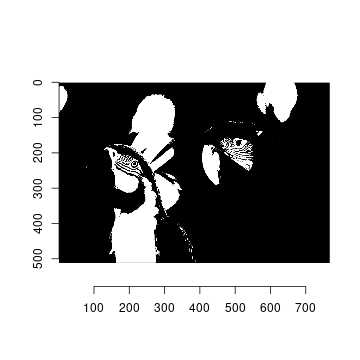Internally, a pixel set is just an array of logical (boolean) values:

``````str(px)
``````
`````` 'pixset' logi [1:768, 1:512, 1, 1] FALSE FALSE FALSE FALSE FALSE FALSE ...
``````

The “TRUE” values correspond to pixels in the set, and “FALSE” to pixels not in the set. The dimensions of the pixset are the same as that of the original image:

``````all(dim(px) == dim(im))
``````
`````` TRUE
``````

To count the number of pixels in the set, use sum:

``````sum(px) #Number of pixels in set
``````
`````` 68993
``````
``````mean(px) #Proportion
``````
`````` 0.1754583
``````

Converting a pixset to an image results in an image of the same size with zeroes and ones:

``````as.cimg(px)
``````
``````Image. Width: 768 pix Height: 512 pix Depth: 1 Colour channels: 1
``````
``````##same thing: automatic conversion to a numeric type
px + 0
``````
``````Image. Width: 768 pix Height: 512 pix Depth: 1 Colour channels: 1
``````

# Indexing using pixsets

You can use pixsets the same way you'd normally use an array of logicals, e.g. for indexing:

``````mean(im[px])
``````
`````` 0.7612596
``````
``````mean(im[!px])
``````
`````` 0.3587848
``````
``````which(px) %>% head
``````
`````` 588 589 590 591 592 593
``````

# Plotting and visualising pixsets

The “highlight” function is a good way of visualising pixel sets:

``````plot(im)
px <- (isoblur(im,4)  > .5 )
highlight(px)
``````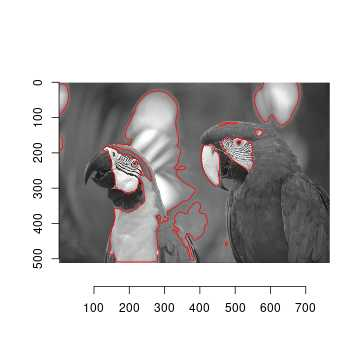highlight extracts the contours of the pixset (see ?contours) and plots them.

colorise is also useful:

``````colorise(im,px,"red",alpha=.5) %>% plot
``````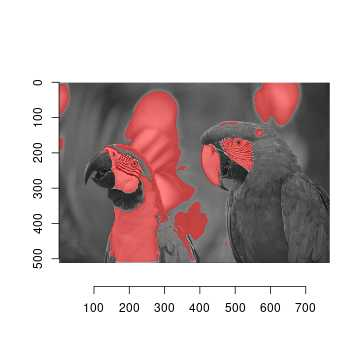You can also use plain old “plot”:

``````plot(px)
``````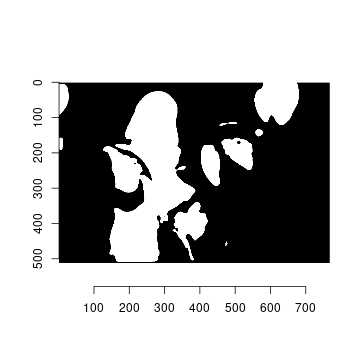It converts “px” to an image and uses plot.cimg.

# Coordinates for pixels in pixsets

The where function returns coordinates for pixels in the set:

``````where(px) %>% head
``````
``````    x y
1 580 1
2 581 1
3 582 1
4 583 1
5 584 1
6 585 1
``````

where returns a data.frame. That format is especially convenient if you want to compute some statistics on the coordinates, e.g., the center of mass of a region defined by a pixset:

``````where(px) %>% dplyr::summarise(mx=mean(x),my=mean(y))
``````
``````        mx       my
1 323.3715 244.2327
``````

# Selecting contiguous regions, splitting into contiguous regions

In segmentation problems one usually wants contiguous regions: px.flood uses the flood fill algorithm (AKA the bucket tool in image editors) to select pixels based on similarity.

``````plot(im)
#Start the fill at location (180,274). sigma sets the tolerance
px.flood(im,180,274,sigma=.21) %>% highlight
``````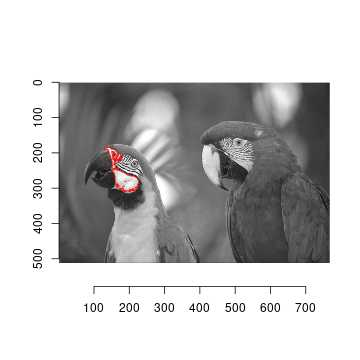It's also common to want to split a pixset into contiguous regions: use split_connected.

``````sp <- split_connected(px) #returns an imlist
plot(sp[1:4])
``````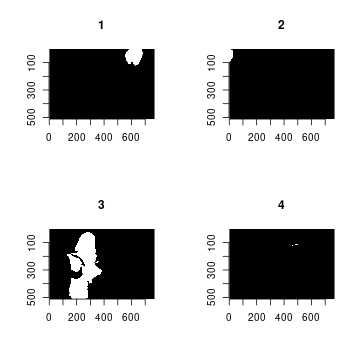``````sp
``````
``````Image list of size 10
``````

Each element in the list is a connected pixset. You can use split_connected to check connectedness (there are faster ways, of course, but this is simple):

``````is.connected <- function(px) length(split_connected(px)) == 1
sapply(sp,is.connected)
``````
``````  TRUE TRUE TRUE TRUE TRUE TRUE TRUE TRUE TRUE TRUE
``````
``````is.connected(px)
``````
`````` FALSE
``````

Use the “high_connectivity” argument to extend to diagonal neighbours as well. See ?label for more.

# Boundaries

The boundary function computes the boundaries of the set:

``````boundary(px) %>% plot
``````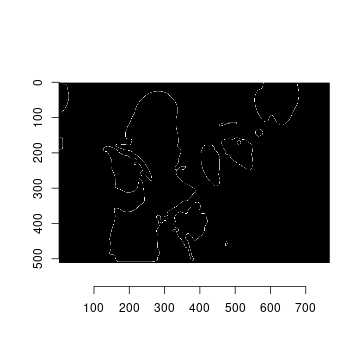``````##Make your own highlight function:
plot(im)
boundary(px) %>% where %\$% { points(x,y,cex=.1,col="red") }
``````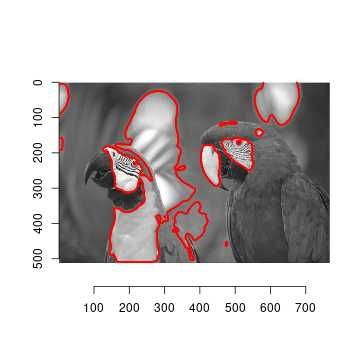# Growing, shrinking, morphological operations

The grow and shrink operators let you grow and shrink pixsets using morphological operators (dilation and erosion, resp.). Have a look at the article on (morphology)[https://dahtah.github.io/imager/morphology.html] for more:

``````plot(im)
highlight(px)
#Grow by 5 pixels
grow(px,5) %>% highlight(col="green")
#Shrink by 5 pixels
shrink(px,5) %>% highlight(col="blue")

#Compute bounding box
bbox(px) %>% highlight(col="yellow")
``````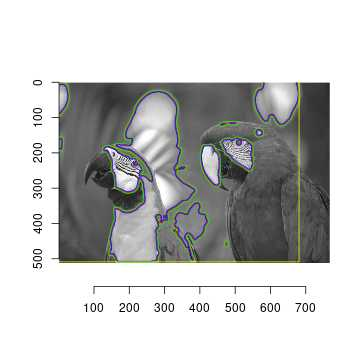# Common pixsets

There's a few convenience functions defining convenience pixsets:

``````px.none(im) #No pixels
``````
``````Pixel set of size 0. Width: 768 pix Height: 512 pix Depth: 1 Colour channels: 1
``````
``````px.all(im) #All of them
``````
``````Pixel set of size 393216. Width: 768 pix Height: 512 pix Depth: 1 Colour channels: 1
``````
``````plot(im)
#Image borders at depth 10
px.borders(im,10) %>% highlight
px.left(im,5) %>% highlight(col="green")
``````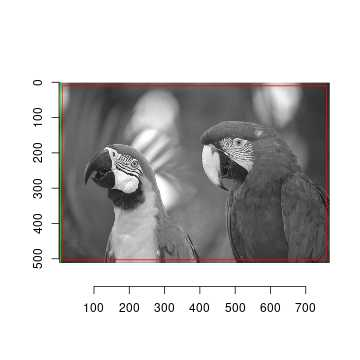# Splitting and concatenating pixsets

imsplit and imappend both work on pixsets.

``````#Split pixset in two along x
imsplit(px,"x",2) %>% plot(layout="row")
``````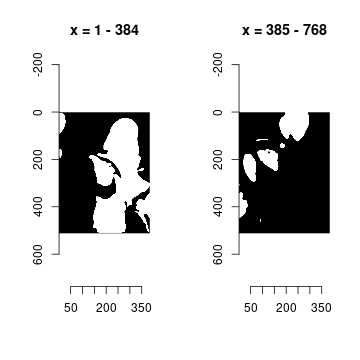``````#Splitting pixsets results into a list of pixsets
imsplit(px,"x",2) %>% str
``````
``````List of 2
\$ x = 1 - 384  : 'pixset' logi [1:384, 1:512, 1, 1] FALSE FALSE FALSE FALSE FALSE FALSE ...
\$ x = 385 - 768: 'pixset' logi [1:384, 1:512, 1, 1] FALSE FALSE FALSE FALSE FALSE FALSE ...
- attr(*, "class")= chr [1:2] "imlist" "list"
``````
``````#Cut along y, append along x
imsplit(px,"y",2) %>% imappend("x") %>% plot()
``````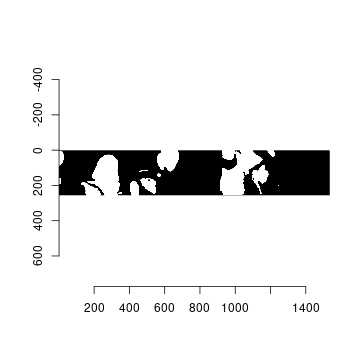You can use reductions the same way you'd use them on images, which is especially useful when working with colour images.

# Working with colour images

Be careful: each colour channel is treated as having its own set of pixels, so that a colour pixset has the same dimension as the image it originated from, e.g.:

``````px <- boats > .8
px
``````
``````Pixel set of size 5650. Width: 256 pix Height: 384 pix Depth: 1 Colour channels: 3
``````
``````where(px) %>% head
``````
``````    x   y cc
1 136 113  1
2 134 114  1
3 143 114  1
4 134 115  1
5 144 115  1
6 133 116  1
``````

Here “px” tells us the location in all locations and across channels of pixels with values higher than .8. If you plot it you'll see the following:

``````plot(px)
``````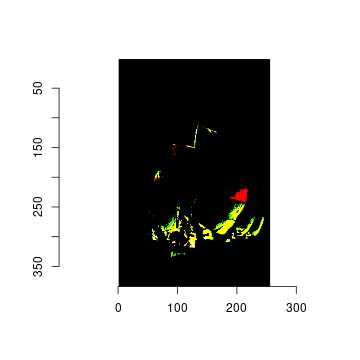Red dots correspond to pixels in the red channel, green in the green channel, etc. You can also view the set by splitting:

``````imsplit(px,"c") %>% plot
``````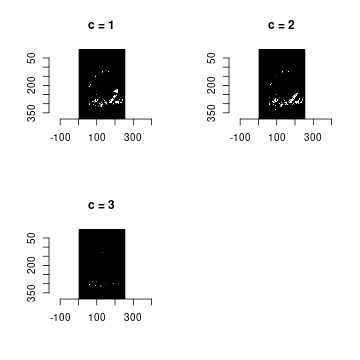If you need to find the pixel locations that have a value of .9 in all channels, use a reduction:

``````#parall stands for "parallel-all", and works similarly to parmax, parmin, etc.
imsplit(px,"c") %>% parall %>% where %>% head
``````
``````    x   y
1 131 126
2 131 127
3 131 128
4 131 129
5 130 131
6 130 132
``````
``````#at each location, test if any channel is in px
imsplit(px,"c") %>% parany %>% where %>% head
``````
``````    x   y
1 135 112
2 136 113
3 134 114
4 143 114
5 134 115
6 144 115
``````
``````#highlight the set (unsurprisingly, it's mostly white pixels)
plot(boats)
imsplit(px,"c") %>% parany %>% highlight
``````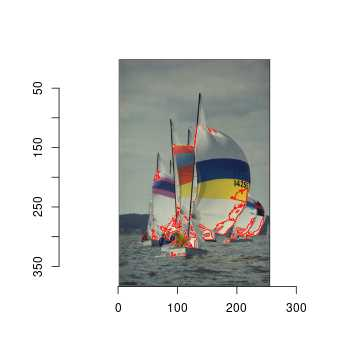# An example: segmentation with pixsets

The following example is derived from the documentation for scikit-image. The objective is to segment the coins from the background.

``````im <- load.example("coins")
plot(im)
``````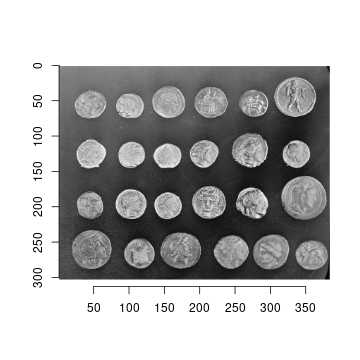A simple thresholding doesn't work because the illumination varies:

``````threshold(im) %>% plot
``````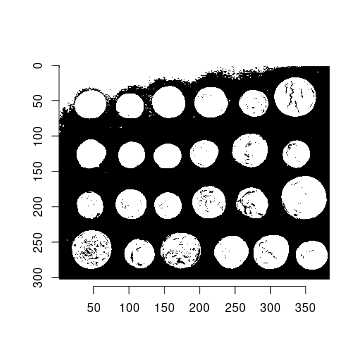It's possible to correct the illumination using a linear model:

``````library(dplyr)
d <- as.data.frame(im)
##Subsamble, fit a linear model
m <- sample_n(d,1e4) %>% lm(value ~ x*y,data=.)
##Correct by removing the trend
im.c <- im-predict(m,d)
out <- threshold(im.c)
plot(out)
``````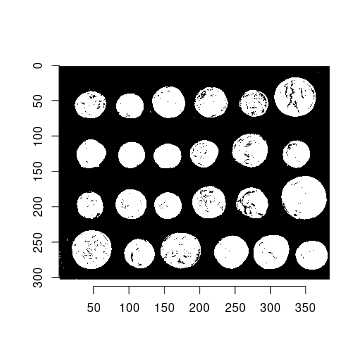Although that's much better we need to clean this up a bit:

``````out <- clean(out,3) %>% imager::fill(7)
plot(im)
highlight(out)
``````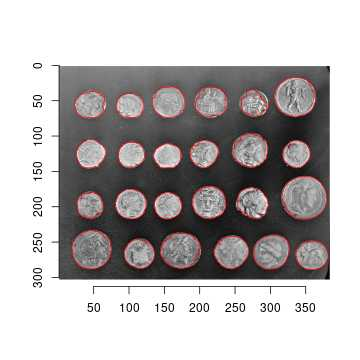Another approach is to use a watershed. We start from seeds regions representing known foreground and known background pixels:

``````bg <- (!threshold(im.c,"10%"))
fg <- (threshold(im.c,"90%"))
imlist(fg,bg) %>% plot(layout="row")
``````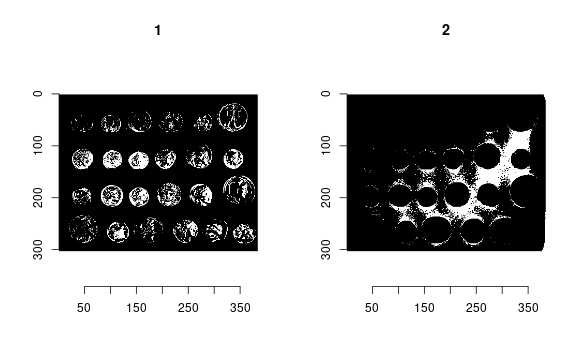``````#Build a seed image where fg pixels have value 2, bg 1, and the rest are 0
seed <- bg+2*fg
plot(seed)
``````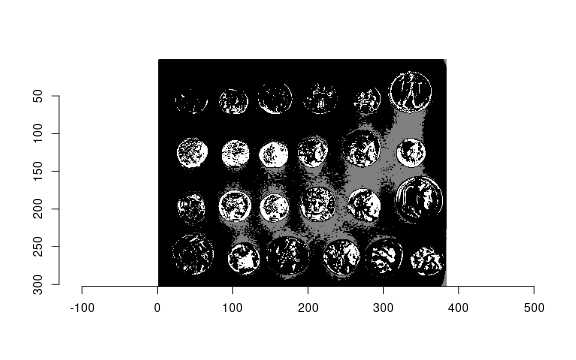The watershed transform will propagate background and foreground labels to neighbouring pixels, according to a priority map (the lower the priority, the slower the propagation). Using the priority map it's possible to prevent label propagation across image edges:

``````edges <- imgradient(im,"xy") %>% enorm
p <- 1/(1+edges)
plot(p)
``````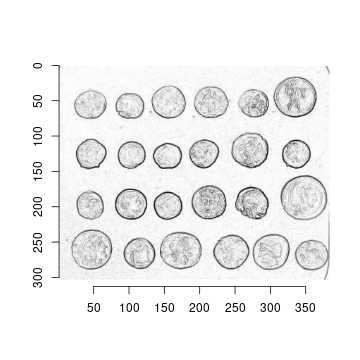We run the watershed transform:

``````ws <- (watershed(seed,p)==1)
plot(ws)
``````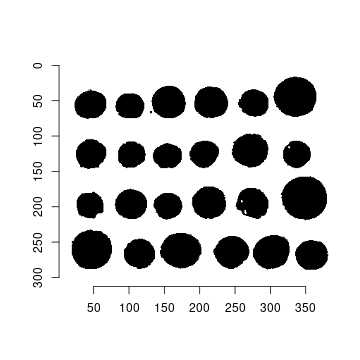We still need to fill in some holes and remove a spurious area. To fill in holes, we use a bucket fill on the background starting from the top-left corner:

``````ws <- bucketfill(ws,1,1,color=2) %>% {!( . == 2) }
plot(ws)
``````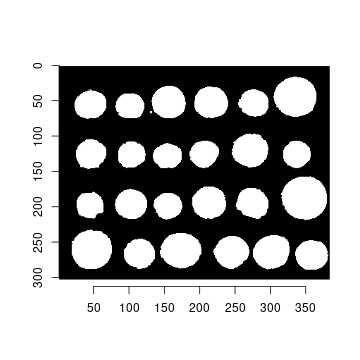To remove the spurious area one possibility is to use “clean”:

``````clean(ws,5) %>% plot
``````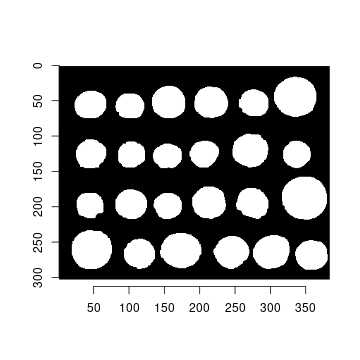Another is to split the pixset into connected components, and remove ones with small areas:

``````split_connected(ws) %>% purrr::discard(~ sum(.) < 100) %>%
parany %>% plot
``````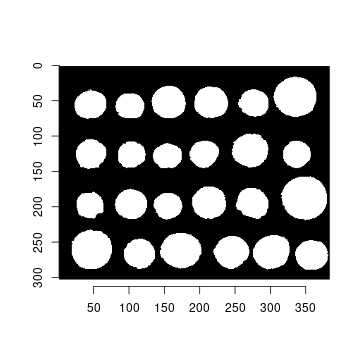Here's a comparison of the segmentations obtained using the two methods:

``````layout(t(1:2))
plot(im,main="Thresholding")
highlight(out)

plot(im,main="Watershed")
out2 <- clean(ws,5)
highlight(out2,col="green")
``````